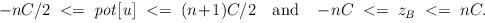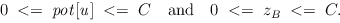Next: Stable Matching ( stable_matching Up: Graph Algorithms Previous: Maximum Cardinality Matchings in   Contents   Index

Subsections

# General Weighted Matchings ( mw_matching )

We give functions

• to compute maximum-weight matchings,
• to compute maximum-weight or minimum-weight perfect matchings, and
• to check the optimality of weighted matchings
in general graph.

You may skip the following subsections and restrict on reading the function signatures and the corresponding comments in order to use these functions. If you are interested in technical details, or if you would like to ensure that the input data is well chosen, or if you would like to know the exact meaning of all output parameters, you should continue reading.

The functions in this section are template functions. It is intended that in the near future the template parameter NT can be instantiated with any number type. Please note that for the time being the template functions are only guaranteed to perform correctly for the number type int. In order to use the template version of the function the appropriate .h-file must be included.

#include <LEDA/graph/templates/mw_matching.h>

There are pre-instantiations for the number types int. In order to use them either

#include <LEDA/graph/mw_matching.h>

or

#include <LEDA/graph/graph_alg.h>

has to be included (the latter file includes the former). The connection between template functions and pre-instantiated functions is discussed in detail in the section Templates for Network Algorithms'' of the LEDA book. The function names of the pre-instantiated versions and the template versions only differ by an additional suffix _T in the names of the latter ones.

#### Proof of Optimality.

Most of the functions for computing maximum or minimum weighted matchings provide a proof of optimality in the form of a dual solution represented by pot, BT and b. We briefly discuss their semantics: Each node is associated with a potential which is stored in the node array pot. The array BT (type array<two_tuple<NT, int> >) is used to represent the nested family of odd cardinality sets which is constructed during the course of the algorithm. For each (non-trivial) blossom B, a two tuple (zB, pB) is stored in BT, where zB is the potential and pB is the parent index of B. The parent index pB is set to -1 if B is a surface blossom. Otherwise, pB stores the index of the entry in BT corresponding to the immediate super-blossom of B. The index range of BT is [0,..., k - 1], where k denotes the number of (non-trivial) blossoms. Let B' be a sub-blossom of B and let the corresponding index of B' and B in BT be denoted by i' and i, respectively. Then, i' < i. In b (type node_array<int>) the parent index for each node u is stored (-1 if u is not contained in any blossom).

#### Heuristics for Initial Matching Constructions.

Each function can be asked to start with either an empty matching ( heur = 0), a greedy matching ( heur = 1) or an (adapted) fractional matching ( heur = 2); by default, the fractional matching heuristic is used.

#### Graph Structure.

All functions assume the underlying graph (type graph) to be connected, simple, and loopfree. They work on the underlying undirected graph of the directed graph parameter.

#### Edge Weight Restrictions.

The algorithms use divisions. In order to avoid rounding errors for the number type int, please make sure that all edge weights are multiples of 4; the algorithm will automatically multiply all edge weights by 4 if this condition is not met. (Then, however, the returned dual solution is valid only with respect to the modified weight function.) Moreover, in the maximum-weight (non-perfect) matching case all edge weights are assumed to be non-negative.

#### Arithmetic Demand.

The arithmetic demand for integer edge weights is as follows. Let C denote the maximal absolute value of any edge weight and let n be the number of nodes of the graph.
In the perfect weighted matching case we have for a potential pot[u] of a node u and for a potential zB of a blossom B:In the non-perfect matching case we have for a potential pot[u] of a node u and for a potential zB of a blossom B:The function CHECK_WEIGHTS may be used to test whether the edge weights are feasible or not. It is automatically called at the beginning of each of the algorithms provided in this chapter.

#### Single Tree vs. Multiple Tree Approach:

All functions can either run a single tree approach or a multiple tree approach. In the single tree approach, one alternating tree is grown from a free node at a time. In the multiple tree approach, multiple alternating trees are grown simultaneously from all free nodes. On large instances, the multiple tree approach is significantly faster and therefore is used by default. If #define _SST_APPROACH is defined before the template file is included all functions will run the single tree approach.

#### Worst-Case Running Time:

All functions for computing maximum or minimum weighted (perfect or non-perfect) matchings guarantee a running time of O(nm log n), where n and m denote the number of nodes and edges, respectively.

 template list MAX_WEIGHT_MATCHING_T(const graph& G, const edge_array& w, bool check = true, int heur = 2) computes a maximum-weight matching M of the underlying undirected graph of graph G with weight function w. If check is set to true, the optimality of M is checked internally. The heuristic used for the construction of an initial matching is determined by heur. Precondition All edge weights must be non-negative. template list MAX_WEIGHT_MATCHING_T(const graph& G, const edge_array& w, node_array& pot, array >& BT, node_array& b, bool check = true, int heur = 2) computes a maximum-weight matching M of the underlying undirected graph of graph G with weight function w. The function provides a proof of optimality in the form of a dual solution given by pot, BT and b. If check is set to true, the optimality of M is checked internally. The heuristic used for the construction of an initial matching is determined by heur. Precondition All edge weights must be non-negative. template bool CHECK_MAX_WEIGHT_MATCHING_T(const graph& G, const edge_array& w, const list& M, const node_array& pot, const array >& BT, const node_array& b) checks if M together with the dual solution represented by pot, BT and b are optimal. The function returns true if M is a maximum-weight matching of G with weight function w. template list MAX_WEIGHT_PERFECT_MATCHING_T(const graph& G, const edge_array& w, bool check = true, int heur = 2) computes a maximum-weight perfect matching M of the underlying undirected graph of graph G and weight function w. If G contains no perfect matching the empty set of edges is returned. If check is set to true, the optimality of M is checked internally. The heuristic used for the construction of an initial matching is determined by heur. template list MAX_WEIGHT_PERFECT_MATCHING_T(const graph& G, const edge_array& w, node_array& pot, array >& BT, node_array& b, bool check = true, int heur = 2) computes a maximum-weight perfect matching M of the underlying undirected graph of graph G with weight function w. If G contains no perfect matching the empty set of edges is returned. The function provides a proof of optimality in the form of a dual solution given by pot, BT and b. If check is set to true, the optimality of M is checked internally. The heuristic used for the construction of an initial matching is determined by heur. template bool CHECK_MAX_WEIGHT_PERFECT_MATCHING_T(const graph& G, const edge_array& w, const list& M, const node_array& pot, const array >& BT, const node_array& b) checks if M together with the dual solution represented by pot, BT and b are optimal. The function returns true iff M is a maximum-weight perfect matching of G with weight function w. template list MIN_WEIGHT_PERFECT_MATCHING_T(const graph& G, const edge_array& w, bool check = true, int heur = 2) computes a minimum-weight perfect matching M of the underlying undirected graph of graph G with weight function w. If G contains no perfect matching the empty set of edges is returned. If check is set to true, the optimality of M is checked internally. The heuristic used for the construction of an initial matching is determined by heur. template list MIN_WEIGHT_PERFECT_MATCHING_T(const graph& G, const edge_array& w, node_array& pot, array >& BT, node_array& b, bool check = true, int heur = 2) computes a minimum-weight perfect matching M of the underlying undirected graph of graph G with weight function w. If G contains no perfect matching the empty set of edges is returned. The function provides a proof of optimality in the form of a dual solution given by pot, BT and b. If check is set to true, the optimality of M is checked internally. The heuristic used for the construction of an initial matching is determined by heur. template bool CHECK_MIN_WEIGHT_PERFECT_MATCHING_T(const graph& G, const edge_array& w, const list& M, const node_array& pot, const array >& BT, const node_array& b) checks if M together with the dual solution represented by pot, BT and b are optimal. The function returns true iff M is a minimum-weight matching of G with weight function w. template bool CHECK_WEIGHTS_T(const graph& G, edge_array& w, bool perfect) returns true, if w is a feasible weight function for G; false otherwise. perfect must be set to true in the perfect matching case; otherwise it must be set to false. If the edge weights are not multiplicatives of 4 all edge weights will be scaled by a factor of 4. The modified weight function is returned in w then. This function is automatically called by each of the maximum weighted machting algorithms provided in this chapter, the user does not have to take care of it.Next: Stable Matching ( stable_matching Up: Graph Algorithms Previous: Maximum Cardinality Matchings in   Contents   Index